• 首先，对于普通的加法器，一般为串行加法器： 构成：把n位全加器串联起来，低位全加器的进位输出连接到相邻的高位全加器的进位输入。...下面介绍本文的重点：超前进位加法器： 设两个数 A , B modu
首先，对于普通的加法器，一般为串行加法器：构成：把n位全加器串联起来，低位全加器的进位输出连接到相邻的高位全加器的进位输入。
特点：进位信号是由低位向高位逐级传递的，进位产生时间较长，且各加法器的和产生时间也不同，速度不高。
分析：该电路的缺点是，对于某一位的运算，需要先进行等待，直到来自低位的进位到达，才能开始本位的计算，因此将会引起较长的时间延迟。所以关键问题就是如何避免等待上一级进位信号，不如提前把进位信号分配给每一位直接运算？
下面介绍本文的重点：超前进位加法器：
设两个数 A , Bmodule fastAdder_4(sum,c_out,a,b,c_in);
//超前进位 四位加法器  代表并行结构
input[3:0] a,b,c_in;
output[3:0] sum,c_out;

wire[3:0] g,p; // g
wire[4:0] c;

assign p=a^b;  	 //传播信号
assign g=a&b;    //生成信号
// 分析 对于某一特定位  是否产生进位
// 取决于两个被加位 | 低位的进位和两个被加位 运算结果相&
assign c=c_in;
assign c=g|(p&c);                         //进位
assign c=g|(p&(g|(p&c)));
assign c=g|(p&(g|(p&(g|(p&c)))));
assign c=g|(p&(g|(p&(g|(p&(g|(p&c)))))));
assign sum = p^c[3:0];
assign c_out=c;
endmodule


测试代码
module fastAdder_4_tb;
reg [3:0] a,b;
reg c_in;
wire [3:0] sum;
wire c_out;
fastAdder_4  U3(.a(a),.b(b),.c_in(c_in),.sum(sum),.c_out(c_out));
initial
begin
a=4'b0001;b=4'b0101;c_in=1'b0;
#100 a=4'b0001;b=4'b0111;
#100 a=4'b0111;b=4'b1100;
#100 b=4'b1111;c_in=1'b1;
#100 a=4'b1101;b=4'b0100;
#100 a=4'b0000;b=4'b1001;c_in=1'b0;
#100 a=4'b1000;b=4'b0110;
end
endmodule


RTL视图：仿真结果如下：第一次写博客，目的是为了做笔记，加深印象，和大家分享我的收获。如果有逻辑不严谨，或者错误之处，大家可以交流一下，一起进步！
补充：
又搞到一个新知识点：关于“流水线超前进位加法器的设计”
关于流水线工作：大家可以去查找相关文档，大致的工作框图如下：可以显著的减少吞吐延时，提高计算效率。
我直接放代码好了：
module fastadder_pipe(sum,c_out,a,b,c_in,clk);
input[3:0] a,b;   input c_in,clk;
output reg[3:0] sum;  output reg c_out;
reg c_out_temp, c_in_temp1,c_in_temp2;
reg[3:0] g,p;reg[4:1] c;

always@(posedge clk)
begin
p<=a^b;
g<=a&b;
end
always@(posedge clk)
begin
c_in_temp1<=c_in;
c_in_temp2<=c_in_temp1;
end
always@(posedge clk)
begin
c=g|(p&c_in_temp1);
c=g|(p&(g|(p&c_in_temp1)));
c=g|(p&(g|(p&(g|(p&c_in_temp1)))));  c=g|(p&(g|(p&(g|(p&(g|(p&c_in_temp1)))))));
end
always@(posedge clk)
sum=p^{c[3:1],c_in_temp2};
always@(posedge clk)
begin
c_out_temp<=c;
c_out<= c_out_temp;
end
endmodule


端口不变 测试文件同上
RTL视图：大家可以观察下，与上一个超前进位的加法器有什么区别，哈哈。


展开全文前端 后端
• 文章目录八位超前进位加法器原理设计文件综合电路测试文件仿真波形八位行波进位加法器原理设计文件方式一综合电路方式二综合电路测试文件仿真波形总结 八位超前进位加法器 原理 有学弟问我，超前进位加法器中的p=...
文章目录八位超前进位加法器原理设计文件综合电路测试文件仿真波形八位行波进位加法器原理设计文件方式一综合电路方式二综合电路测试文件仿真波形总结
八位超前进位加法器
原理

有学弟问我，超前进位加法器中的p=add1 ^ add2和g=add1 & add2是什么意思，所以这篇文章就稍微赘述一点吧~

所谓超前进位，首先应该明白进位是什么。
8+4=12，其中的1就是进位，其中的2才是结果。
那么在二进制中，1+1=10，其中的1就是进位，0就是结果。
明白了进位和结果，那么多位的数字是怎么计算呢？
其实上面的计算应该是1+1+上一位的进位=10，所以两个数字的计算应该涉及三个内容——加数，被加数，上一位的进位。
在一位二进制加法中，a和b分别表示两个加数，cin表示上一位的进位，cout表示下一位的进位，sum表示结果，我们列出其真值表：

add1
add2
cin
cout
sum

0
0
0
0
0

0
1
0
0
1

1
0
0
0
1

1
1
0
1
0

0
0
1
0
1

0
1
1
1
0

1
0
1
1
0

1
1
1
1
1

以数字**1为真**，数字**0为假**，a表示1，a'表示0，这样的形式。学过离散数学或者数字电路的同学应该多少会点吧，“·”代表“与”，“+”代表“或”，所以上面的真值表，写成表达式，就是：
$cout = add1·add2·cin'+add1'·add2·cin+add1·add2'cin+add1·add2·cin$
经过部分化简：
$cout=add1·add2+add1'add2·cin+add1·add2'·cin=add1·add2+cin·(add1⊕add2)$
同理：
$sum =add1⊕add2⊕cin$
找到两个等式的相同部分，都有a⊕b，其中⊕代表“异或”，在Verilog中用“^”表示，即a^b，所以令p = add1 ^ add2，现在知道干什么用的了嘛？
没错，就是中间变量而已，同理g = add1 & add2也是中间变量，这样就可以把上面两个等式改写成：cout = g | (cin & p)和sum = add1 ^ add2 ^ cin（其实就是变短了一点点而已）

不过呢，适当的使用中间变量，可能会让你的代码精简许多~

此外，我发现一个网站可以通过真值表生成表达式：http://www.32x8.com/index.html
设计文件
module add_8_1(
input wire [7:0] add1,
input wire [7:0] add2,
input wire cin,
output wire [7:0] sum,
output wire cout
);
wire[7:0] g,p,c;//g和p的含义已经讲解了，c代表每一位计算的进位，c表示原始的进位cin
assign c=cin;
assign p=add1 ^ add2;
assign g=add1 & add2;
assign c = g | (p & c);
assign c = g | (p & (g | (p & c)));
assign c = g | (p & (g | (p & (g | (p & c)))));
assign c = g | (p & (g | (p & (g | (p & (g | (p & c)))))));
assign c = g | (p & (g | (p & (g | (p & (g | (p & (g | (p & c)))))))));
assign c = g | (p & (g | (p & (g | (p & (g | (p & (g | (p & (g | (p & c)))))))))));
assign c = g | (p & (g | (p & (g | (p & (g | (p & (g | (p & (g | (p & (g | (p & c)))))))))))));
assign c = g | (p & (g | (p & (g | (p & (g | (p & (g | (p & (g | (p & (g | (p & (g | (p & c)))))))))))))));
assign sum=p^c[7:0];
assign cout=c;
endmodule

上面的代码应该看起来很长，像老太太的裹脚布，但这也就是所谓的超前进位。
Verilog中“块”和assign语句都是并行执行的，上面有许多assign语句，所以从c到c，根据第一步的cin，就可以同步计算出每一位的进位（超前进位），以及最后的结果，这些是同时完成的，也就完成了超前进位加法器。
综合电路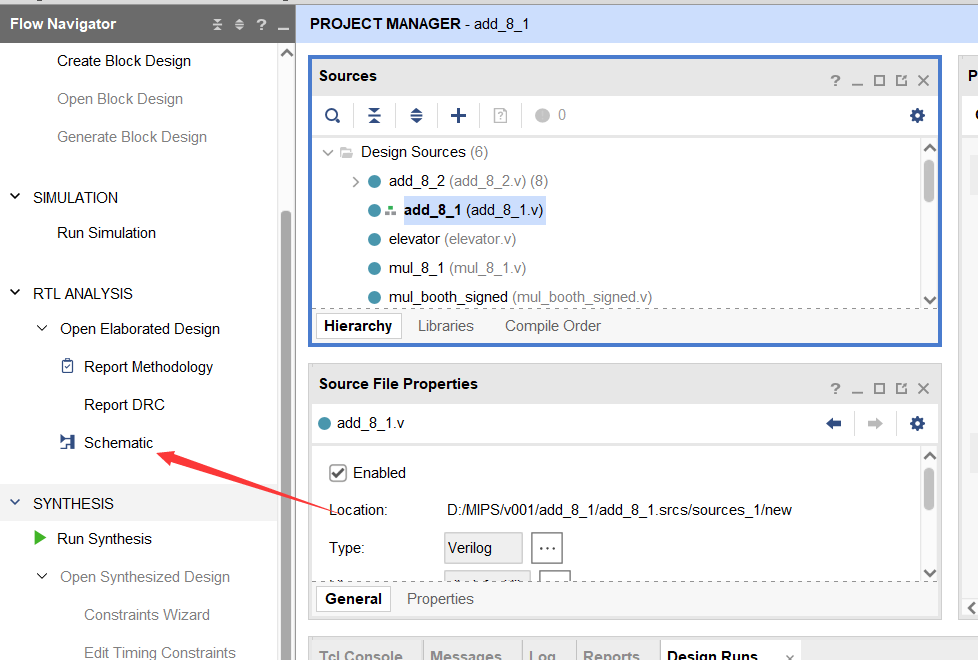点击RTL-ANALYSIS——Synthesis，可以查看综合电路图：
19个元件，26个IO口，56根线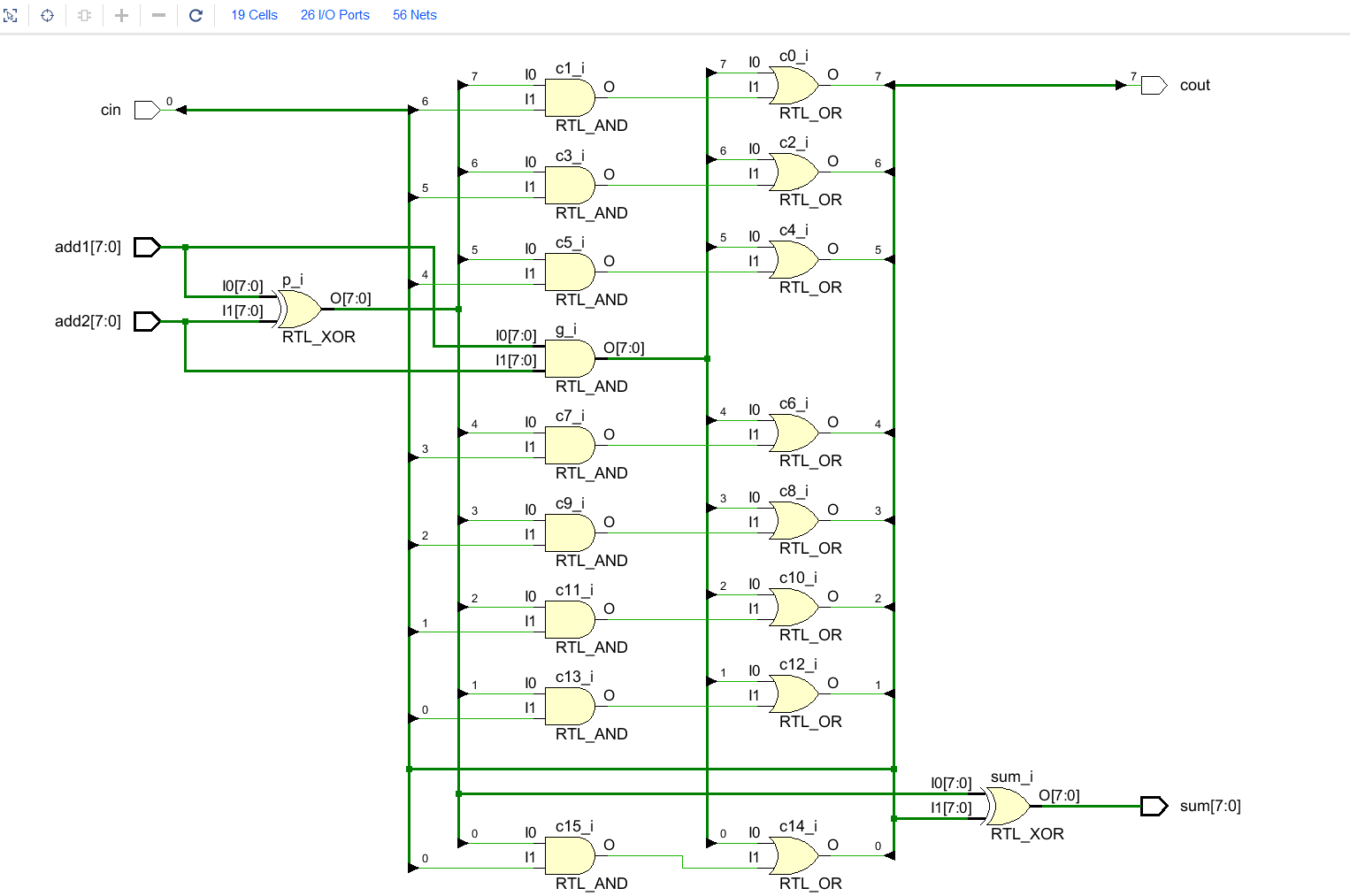测试文件
timescale 1ns / 1ps
module add_tb();
reg [7:0] add1,add2;
reg cin;
reg clk;
wire [7:0] sum;
wire cout;
initial begin
add1 <= 8'd10;
add2 <= 8'd3;
cin <= 1'b0;
clk <= 1'b0;
end

always # 10 clk = ~clk;
always @ (negedge clk) begin
{add1,add2} <= {add1,add2} + 1'b1;
end

add_8_1 try(.add1(add1),.add2(add2),.cin(cin),.sum(sum),.cout(cout));

endmodule

还有些同学，对测试文件的理解也还是不够深刻，这里稍提一嘴，测试文件就是给电路加激励信息，使电路运作。至于加提示信息之类的，各自加油就好啦~
仿真波形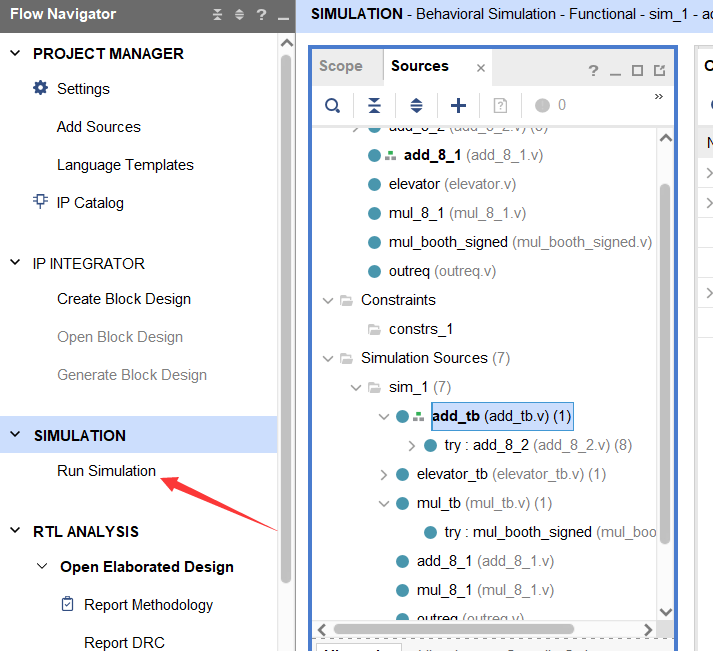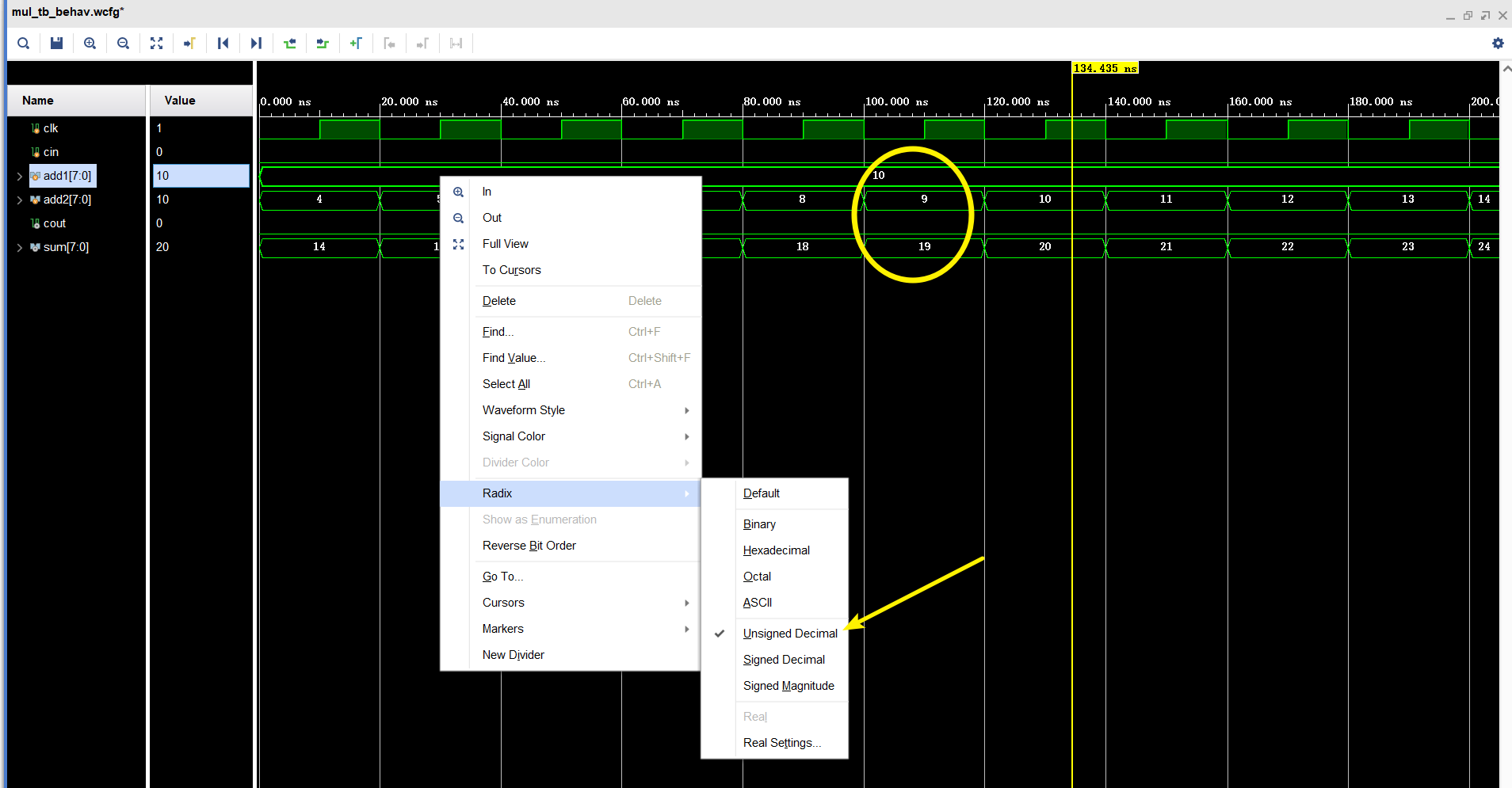右键某一信号，选择相应的进制数，以10+9为例，最终输出cout=0，sum=19
那么之后的10+11为什么没有进位使cout=1呢？
把他们转换成二进制观察一下就明白啦~
八位行波进位加法器

当时不知道在哪听的这个名字，就一直用下了，其实这个应该叫做行波进位加法器——由N个全加器级联而成，所以被我叫成了级联进位emmm

原理
如上所说，行波进位加法器是由N个全加器级联而成，比如8位的加法器可以由8个一位全加器级联而成，也可以由2个四位全加器构成，区别就是所综合出的电路大小。
设计文件
方式一
module add_8_2(
input wire [7:0] add1,add2,
input wire cin,
output reg [7:0] sum,
output reg cout
);
reg[7:0] G,P,C;
always @(add1 or add2 or cin)
begin
G = add1 & add2;
P = add1 ^ add2;
C = cin; //最低位的进位输入，即cin
sum = G^ P ^ C;//第一位的计算结果
G = add1 & add2;
P = add1 ^ add2;
C = G |(P & C);//c=add1add2+(add1+add2)cin=G|(P&cin)
sum = G ^ P ^ C;
G = add1 & add2;
P = add1 ^ add2;
C = G |(P & C);
sum = G ^ P ^ C;
G = add1& add2;
P = add1 ^ add2;
C = G |(P & C);
sum = G ^ P ^ C;
G = add1 & add2;
P = add1 ^ add2;
C = G |(P & C);
sum = G ^ P ^ C;
G = add1 & add2;
P = add1 ^ add2;
C = G |(P & C);
sum = G ^ P ^ C;
G = add1 & add2;
P = add1 ^ add2;
C = G |(P & C);
sum = G ^ P ^ C;
G = add1 & add2;
P = add1 ^ add2;
C = G |(P & C);
sum = G ^ P ^ C;
cout = G |(P & C);
end
endmodule

这是把上述原理复现了一边，上一步的进位输出，传入到下一位计算的进位输入。
综合后的电路如下，48个元件，26个IO口，65根线
综合电路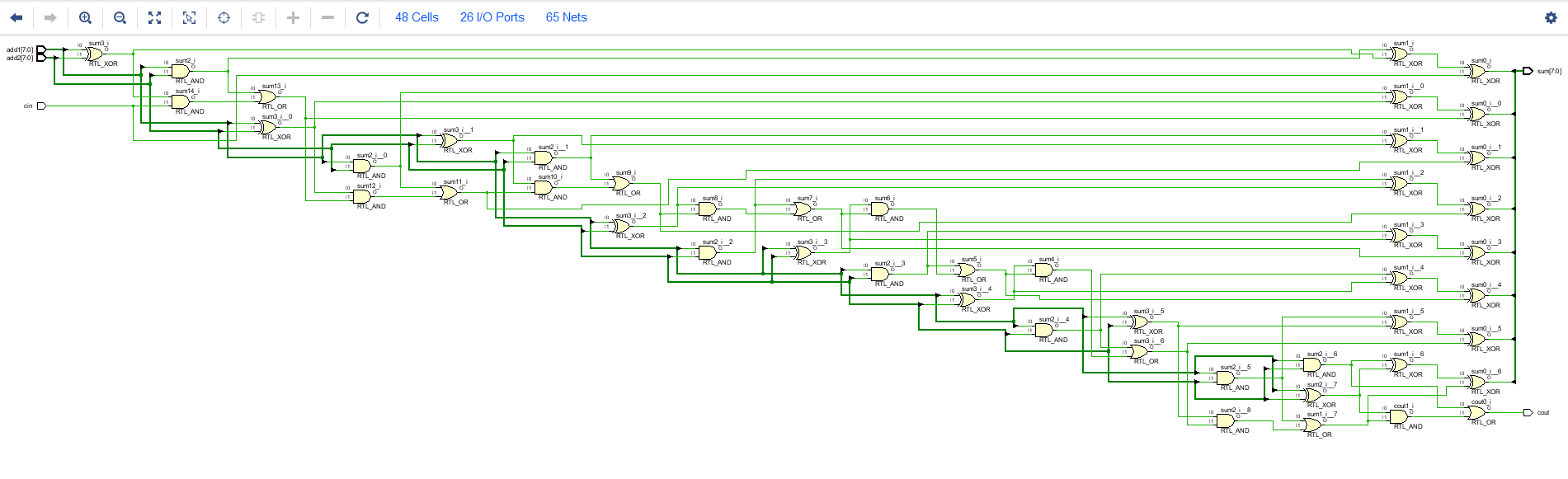方式二
上面的语句重复性太大，就用模块例化的方式来写
module add_8_2(
input wire [7:0] add1,add2,
input wire cin,
output wire [7:0] sum,
output wire cout
);
wire [6:0] c;
add_1_unit a1(add1,add2,cin,c,sum);
add_1_unit a2(add1,add2,c,c,sum);
add_1_unit a3(add1,add2,c,c,sum);
add_1_unit a4(add1,add2,c,c,sum);
add_1_unit a5(add1,add2,c,c,sum);
add_1_unit a6(add1,add2,c,c,sum);
add_1_unit a7(add1,add2,c,c,sum);
add_1_unit a8(add1,add2,c,cout,sum);
endmodule

其中的add_1_unit模块如下：
module add_1_unit(
input wire add1,add2,cin,
output wire cout,sum
);
assign cout = (add1 & add2) | (cin & (add1 ^ add2));
assign sum = add1 ^ add2 ^ cin;
endmodule

综合后的电路如下，全展开后有48个元件，26个IO口，97根线
综合电路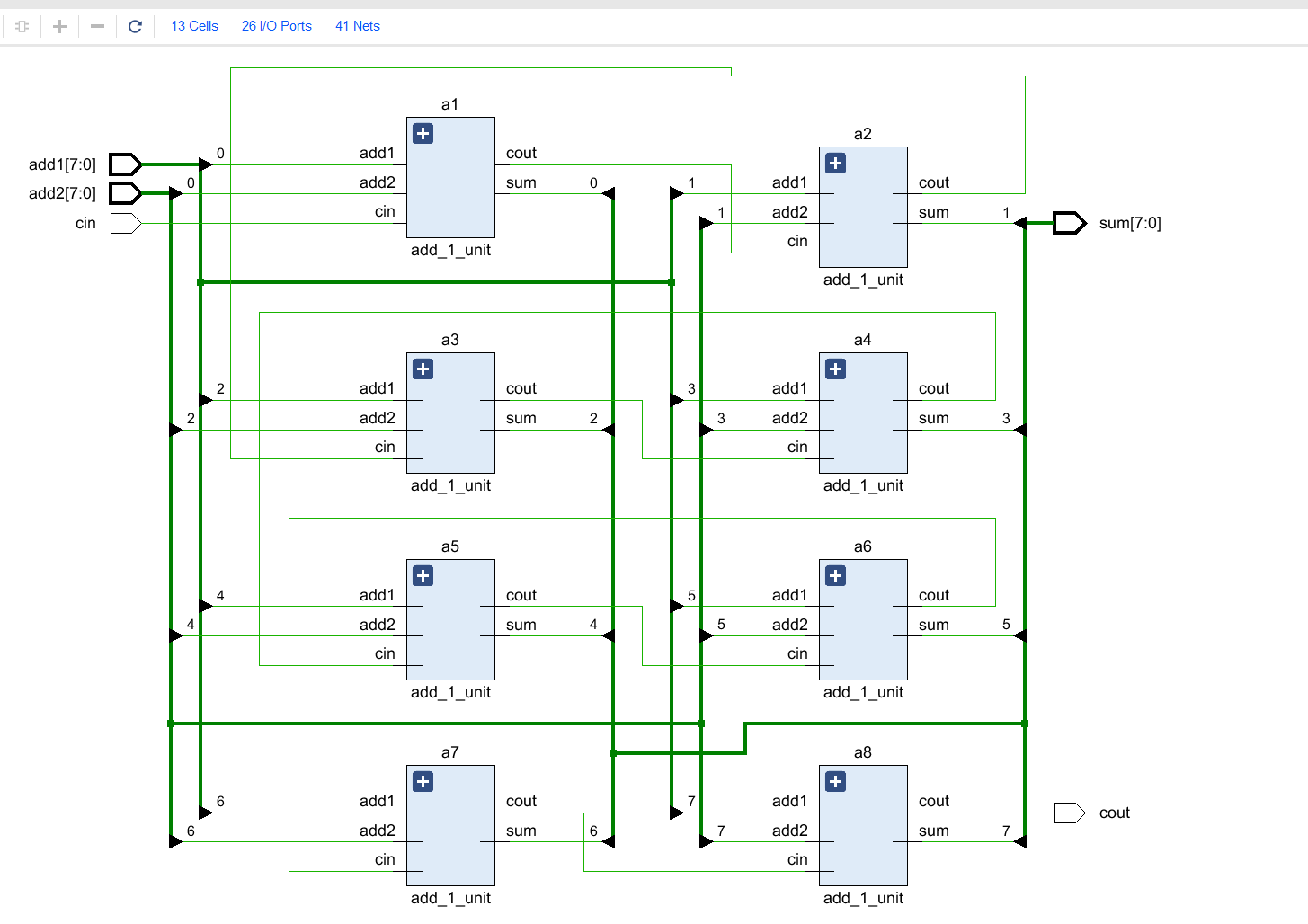其中的每一个单元如下：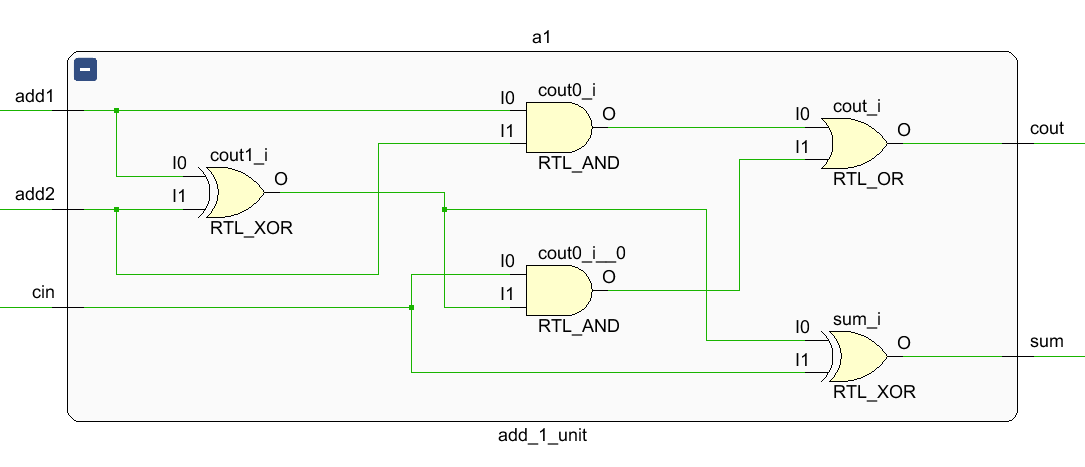全展开后如下：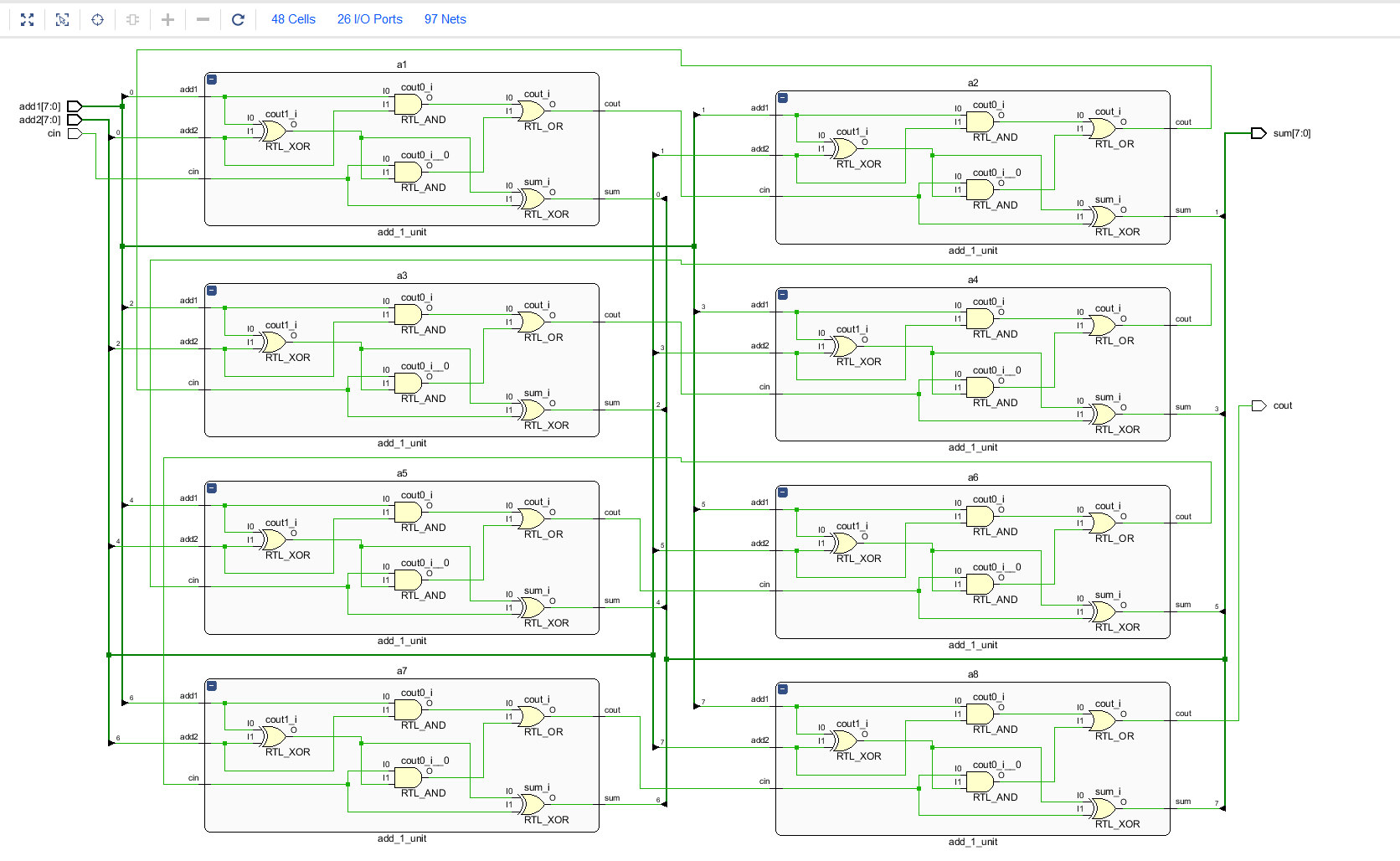两种写法
数据总线的数量有区别：一个是65根线，一个是97根线。
代码量有区别：一个40行左右，一个总共27行左右。
优缺点不言而喻。
测试文件
都是八位加法器，与超前进位加法器也没区别
还是放一下吧：
timescale 1ns / 1ps
module add_tb();
reg [7:0] add1,add2;
reg cin;
reg clk;
wire [7:0] sum;
wire cout;
initial begin
add1 <= 8'd10;
add2 <= 8'd3;
cin <= 1'b0;
clk <= 1'b0;
end

always # 10 clk = ~clk;
always @ (negedge clk) begin
{add1,add2} <= {add1,add2} + 1'b1;
end

add_8_2 try(.add1(add1),.add2(add2),.cin(cin),.sum(sum),.cout(cout));

endmodule

仿真波形
与超前进位一致，用二进制查看一下如下图：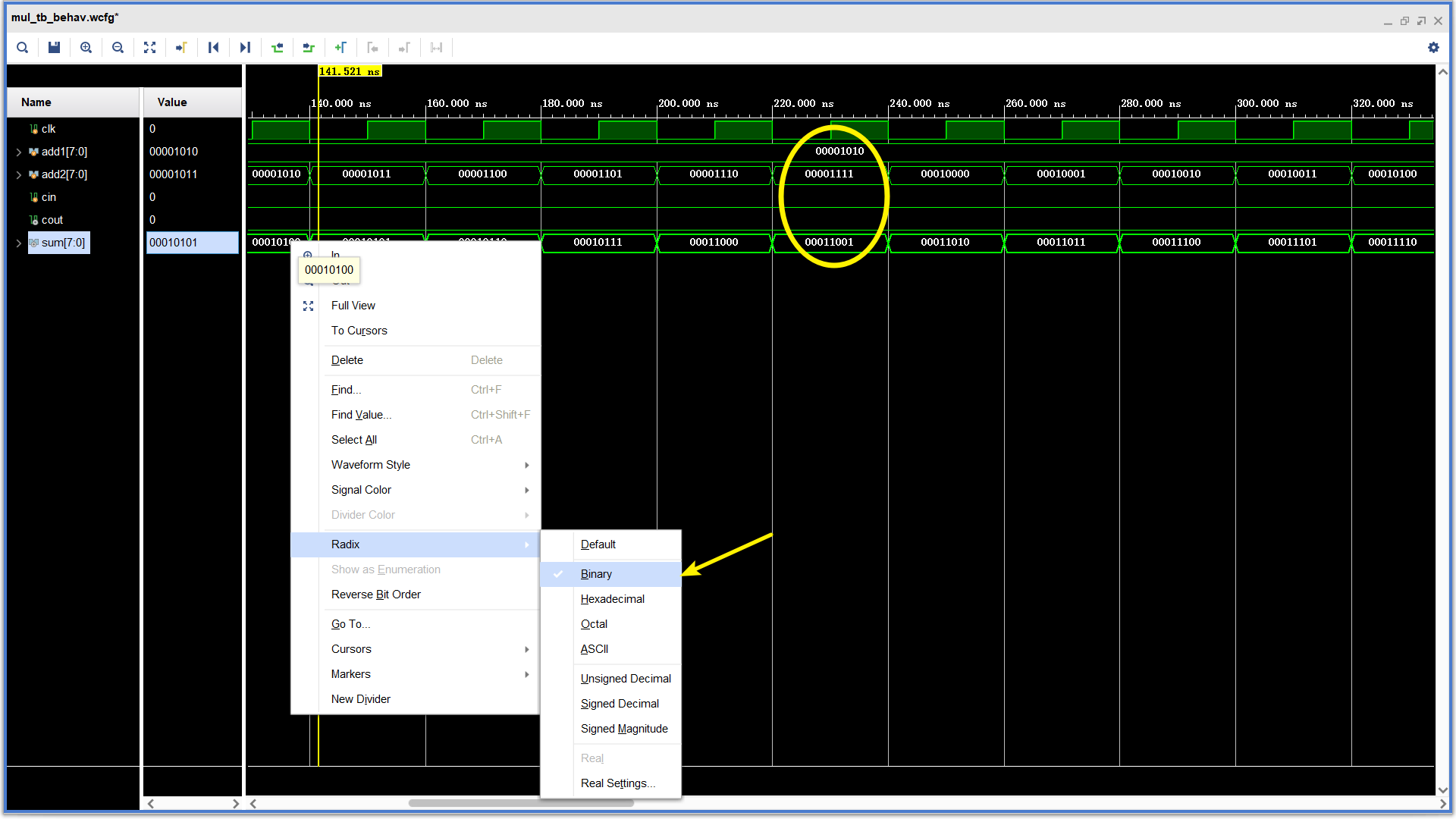总结
行波进位加法器，由于后一位的进位依赖于前一位的进位，所以关键路径更长，限制速度，性能不高，
超前进位加法器，由于进位计算是并行的，所以关键路径短，速度快，但是位宽越宽，也就代表会综合出更复杂的、面积更大的电路。


展开全文fpga verilog
• ## 超前进位加法器（较为详细讲解）

万次阅读 多人点赞 2020-05-17 17:39:57
超前进位加法器
先谈谈超前进位加法器设计的初衷。
我们从数电书学到的最基本的加法器的结构是下图这样的：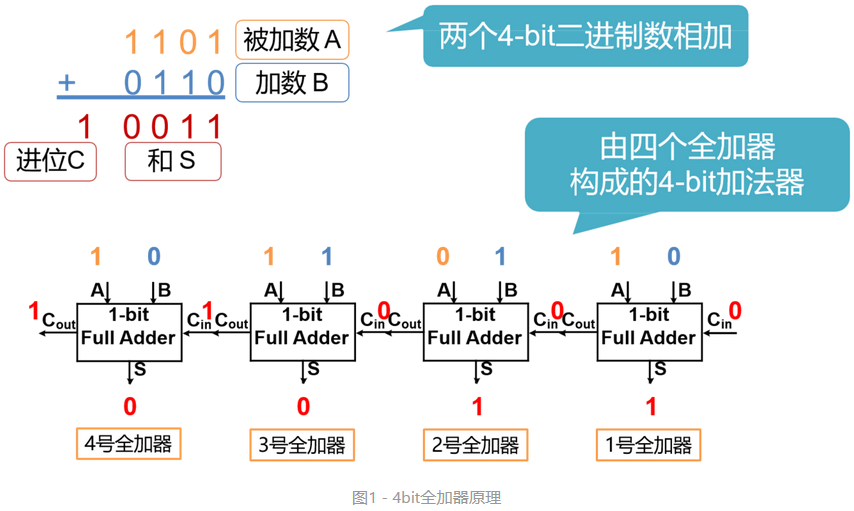从图中很好理解加法器的组成。
一位的加法器直接卡诺图既可以画出来。
但是这样的结构有什么缺点？最直接的就是第4号全加器要输出计算结果至少要等到第3号全加器把进位信息传过来。那如果级数很高会出现组合逻辑延时过长的情况。
下面这篇文章很详细的分析了门级延时的情况。
https://www.jianshu.com
其实问题的关键在于如何解决这个等待进位信息的问题。我们可以想可不可以不等后级把进位算完再传过来，我们直接通过输入的数据把这个进位直接算出来发给每一级不就好了。
我们看看怎么实现这样的想法.
简单的1级加法器的进位信号计算的方式如下所示：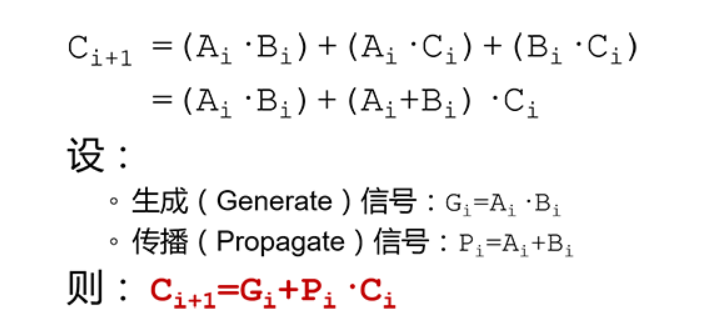那4级的加法器进位信息可以这样表示：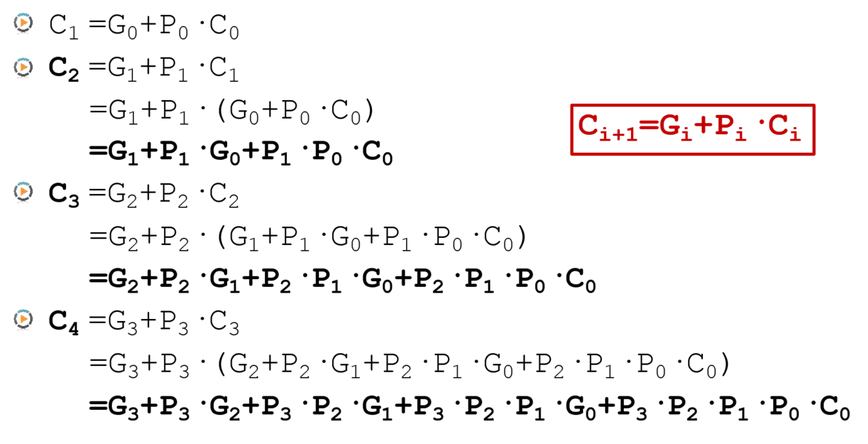//一位加法器
module Add1
(
input a,
input b,
input C_in,
output f,
output g,
output p
);
assign f=a^b^C_in;
assign g=a&b;
assign p=a|b;
endmodule

//4位CLA部件
module CLA_4(
input [3:0]P,
input [3:0]G,
input C_in,
output [4:1]Ci,
output Gm,
output Pm
);
assign Ci=G|P&C_in;
assign Ci=G|P&G|P&P&C_in;
assign Ci=G|P&G|P&P&G|P&P&P&C_in;
assign Ci=G|P&G|P&P&G|P&P&P&G|P&P&P&P&C_in;

assign Gm=G|P&G|P&P&G|P&P&P&G;
assign Pm=P&P&P&P;
endmodule

上面是根据公式写出来的1位加法器和进位计算模块。
但是貌似与传统的加法器不太一样，这里的P和G就是传播信号和生成信号。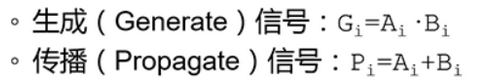总体的的4位超前进位加法器如下图所示：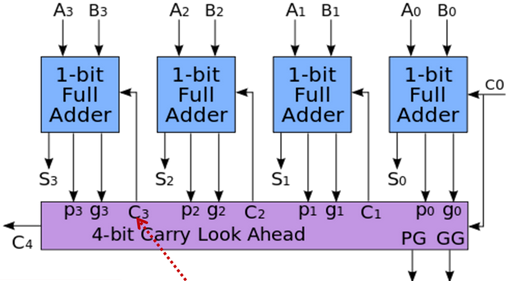//四位超前进位加法器
module Add4_head
(
input [3:0]A,
input [3:0]B,
input C_in,
output [3:0]F,
output Gm,
output Pm,
output C_out
);
wire [3:0] G;
wire [3:0] P;
wire [4:1] C;
Add1 u1
(	.a(A),
.b(B),
.C_in(C_in),
.f(F),
.g(G),
.p(P)
);
Add1 u2
(	.a(A),
.b(B),
.C_in(C),
.f(F),
.g(G),
.p(P)
);
Add1 u3
(	.a(A),
.b(B),
.C_in(C),
.f(F),
.g(G),
.p(P)
);
Add1 u4
(	.a(A),
.b(B),
.C_in(C),
.f(F),
.g(G),
.p(P)
);
CLA_4 uut
(
.P(P),
.G(G),
.C_in(C_in),
.Ci(C),
.Gm(Gm),
.Pm(Pm)
);
assign C_out=C;
endmodule

新的问题来了，4位的好解决，但是16位的，这样直接算进位太复杂了。有没有什么好的办法。
注意到我们这里的4位的加法器把Pm,Gm也引出来了。我们可不可以仿照类似4位的加法器，把1个4位的加法器当成之前的1位加法器，同样是将Pm,Gm这两个用CLA计算进位信息即可。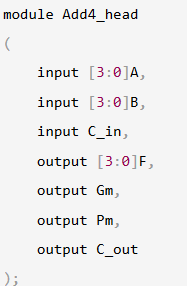//16位
module Add16_head
(
input [15:0]A,
input [15:0]B,
input C_in,
output [15:0] F,
output Gm,
output Pm,
output C_out
);
wire [3:0]G;
wire [3:0]P;
wire [4:1]C;
Add4_head A0
(
.A(A[3:0]),
.B(B[3:0]),
.C_in(C_in),
.F(F[3:0]),
.Gm(G),
.Pm(P)
);
Add4_head A1
(
.A(A[7:4]),
.B(B[7:4]),
.C_in(C),
.F(F[7:4]),
.Gm(G),
.Pm(P)
);
Add4_head A3
(
.A(A[11:8]),
.B(B[11:8]),
.C_in(C),
.F(F[11:8]),
.Gm(G),
.Pm(P)
);
Add4_head A4
(
.A(A[15:12]),
.B(B[15:12]),
.C_in(C),
.F(F[15:12]),
.Gm(G),
.Pm(P)
);
CLA_4 AAt
(
.P(P),
.G(G),
.C_in(C_in),
.Ci(C),
.Gm(Gm),
.Pm(Pm)
);
assign C_out=C;
endmodule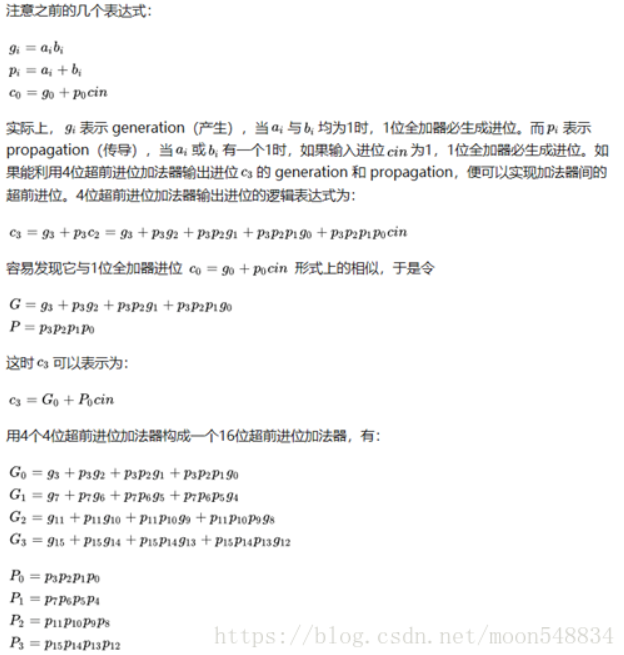所以还是原来的套路。
对于一些比较奇葩的位数，我们可以通过级联4位和16位的加法器组合起来用，
比如24位的可以这样做：
//24位加法
module Add24_head
(
input [23:0]A,
input [23:0]B,
input C_in,
output [23:0]Result,
output C_out
);
wire [3:0]G;wire [3:0]P;
wire [2:1]C;
Add16_head add16_11
(
.A(A[15:0]),
.B(B[15:0]),
.C_in(C_in),
.F(Result[15:0]),
.C_out(C)
);
Add4_head add4_11
(
.A(A[19:16]),
.B(B[19:16]),
.C_in(C),
.F(Result[19:16]),
.C_out(C)
);
Add4_head add4_12
(
.A(A[23:20]),
.B(B[23:20]),
.C_in(C),
.F(Result[23:20]),
.C_out(C_out)
);

endmodule

对于32位的除了级联两个16位的，更优化的是可以参照计算两位加法器的进位运算
 //32位加法
module Add32_head
(
input [31:0]A,
input [31:0]B,
input C_in,
output [31:0]Result,
output C_out
);
wire [3:0]G;wire [3:0]P;
assign G[3:2]=2'b00;
assign P[3:2]=2'b00;
Add16_head add16_1
(
.A(A[15:0]),
.B(B[15:0]),
.C_in(C_in),
.F(Result[15:0]),
.Gm(G),
.Pm(P)
);
wire C_16;
Add16_head add16_2
(
.A(A[31:16]),
.B(B[31:16]),
.C_in(C_16),
.F(Result[31:16]),
.Gm(G),
.Pm(P)
);
assign C_16=G|P&C_in;
assign C_out=G|P&G|P&P&C_in;
endmodule

实际上依然运用的思想还是通过预算出进位信息来达到减少延时的功能。


展开全文•cpu设计
• 此设计是超前进位加法器，包括ise工程，应用于CPU设计之中。FPGA 数电
• 代码准确可靠。4位超前进位加法器的数据流建模。利用Verilog HDL数据流建模方法建立4位超前进位加法器，并完成仿真和综合
• 一、半加器和全加器 二、行波进位加法器 三、超前进位加法器（Carry-Lookahead Adder，CLA） https://www.jianshu.com/p/6ce9cad8b467
一、半加器和全加器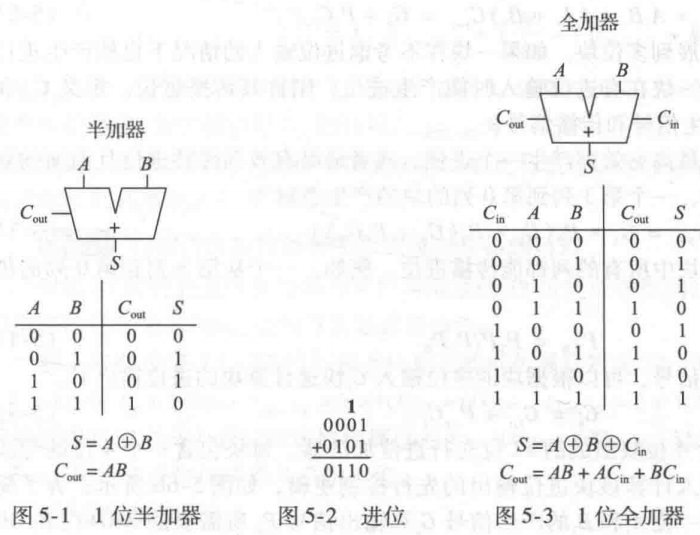二、行波进位加法器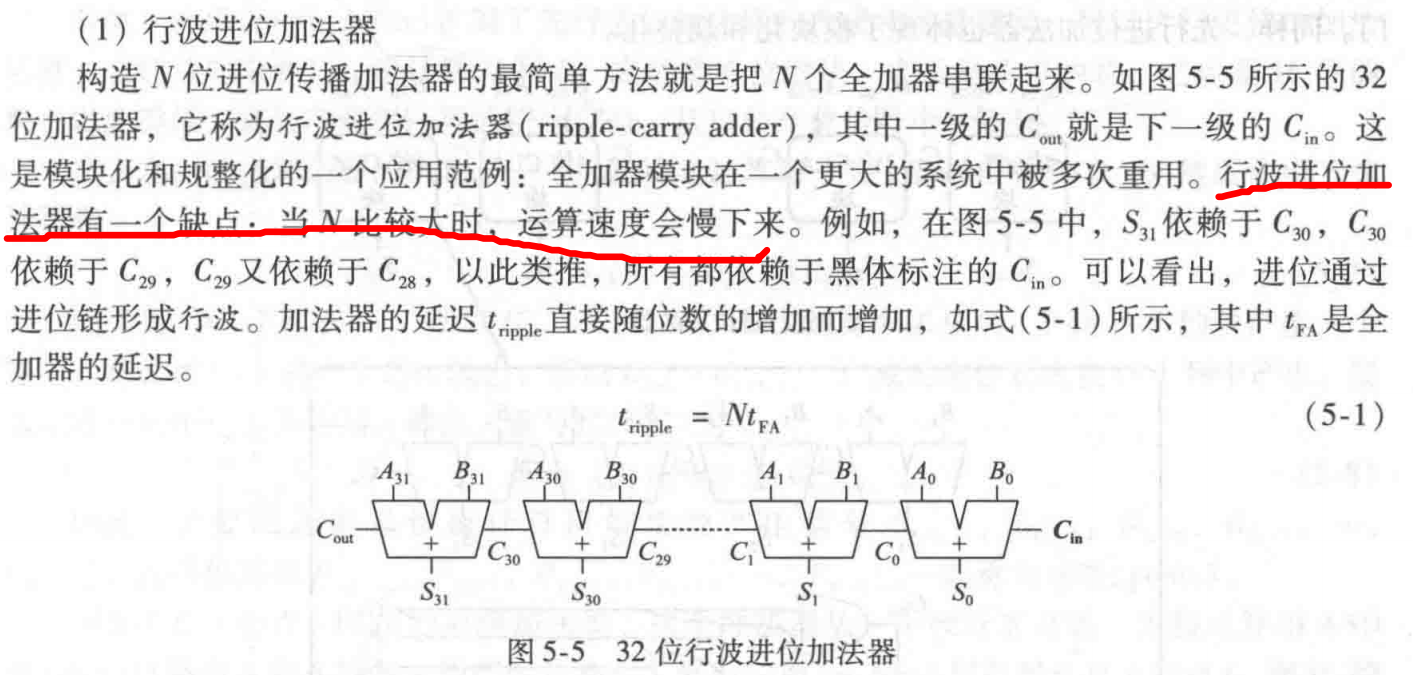三、超前进位加法器（Carry-Lookahead Adder，CLA）

https://www.jianshu.com/p/6ce9cad8b467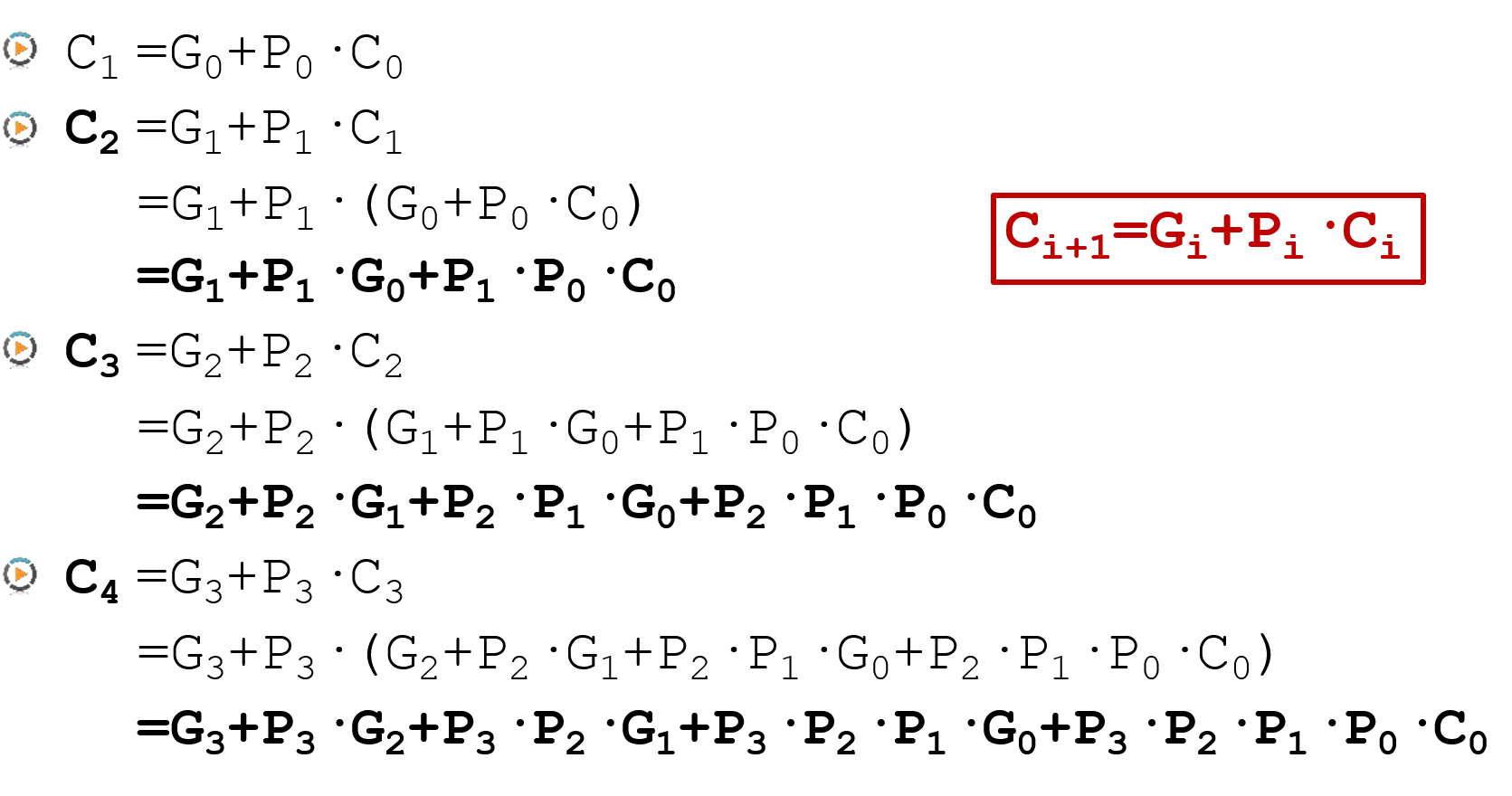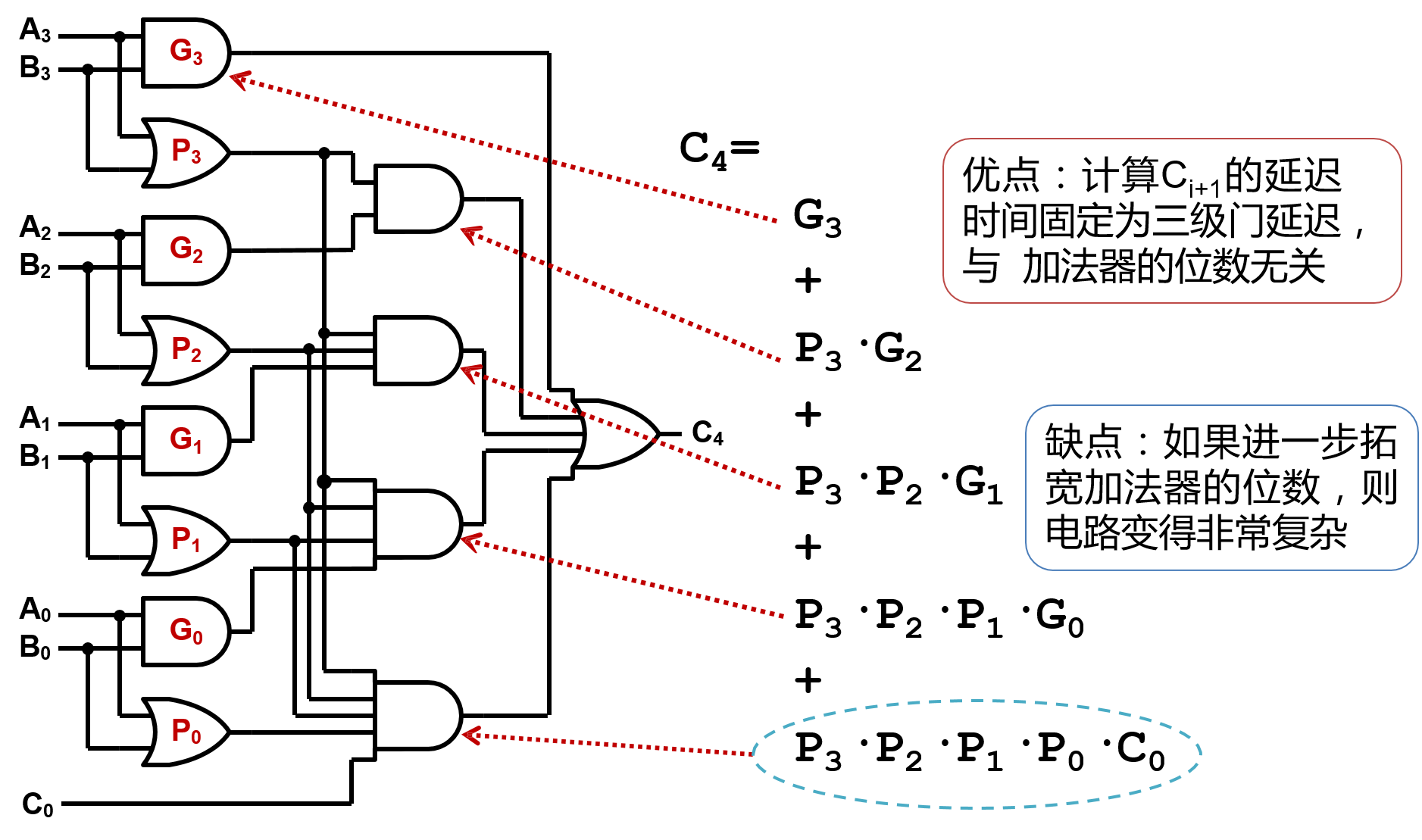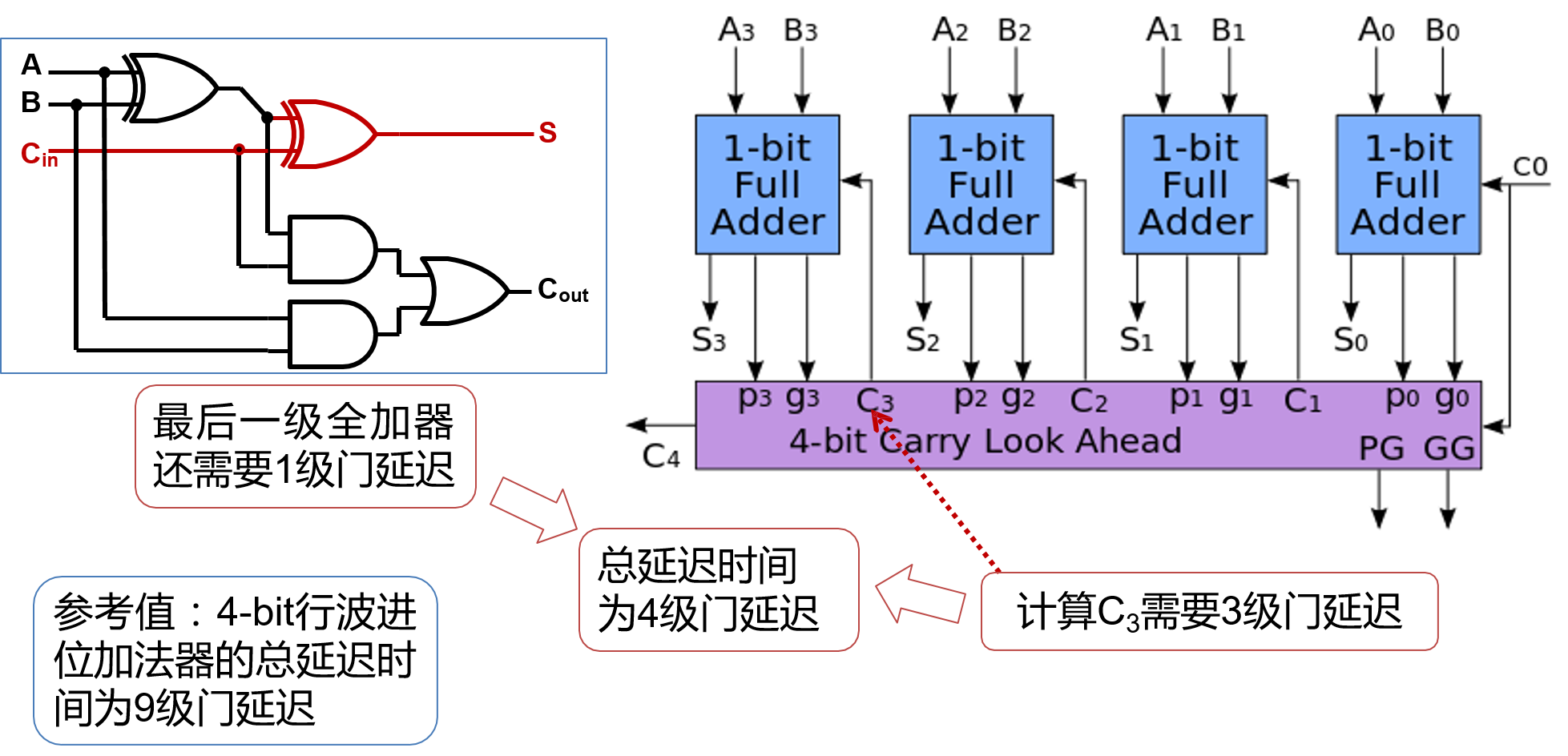四、流水线加法器

源文件：

timescale 1ns / 1ps
module adder_pipeline2(
input clk,
input [7:0]a,
input [7:0]b,
input cin,
output reg[7:0]sum,
output reg cout
);

reg [3:0]tem_a;
reg [3:0]tem_b;
reg [3:0]sum1;
reg cout1;
always@(posedge clk)//第一级流水线
begin
{cout1,sum1}<=a[3:0]+b[3:0]+cin;
end

always@(posedge clk)//将输入寄存一拍
begin
tem_a<=a[7:4];
tem_b<=b[7:4];
end

always@(posedge clk)//第二级流水线
{cout,sum}<={{1'b0,tem_a}+{1'b0,tem_b}+cout1,sum1};
endmodule


测试文件：

timescale 1ns / 1ps
module tb_adder2pipeline;
reg clk;
reg [7:0]a;
reg [7:0]b;
reg cin;
wire [7:0]sum;
wire cout;
initial
clk=0;
always #10clk=~clk;

always@(posedge clk)begin
a<={$random}%255; b<=2+{$random}%250;
cin<={\$random}%2;
end

adder_pipeline2 u_adder(
.clk(clk),
.a(a),
.b(b),
.cin(cin),
.cout(cout),
.sum(sum)
);
endmodule


仿真波形：

两级流水线，加法器的和延迟两个周期输出结果。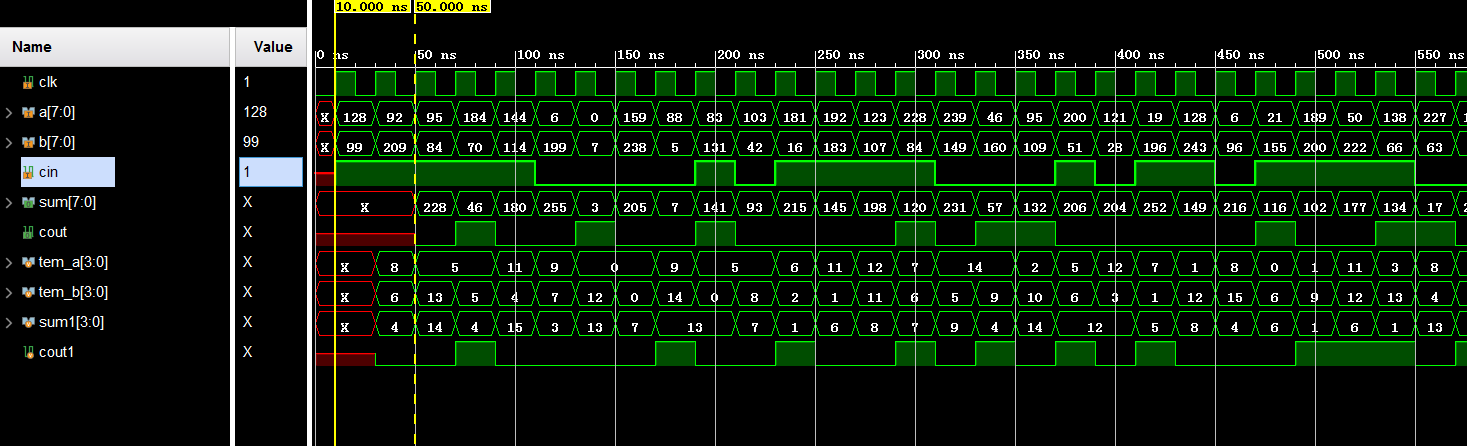展开全文• 超前进位加法器verilog代码 verilog代码 , 超前进位
• 参考4位超前进位加法器的8位超前进位加法器数据流建模+层次建模，试用Verilog HDL对其进行建模，有测试文件，代码准确无错。
• 4bit超前进位加法器（CLA）源代码，用组合逻辑实现
• 仿真结果表明，在SMIC0.18μm工艺下，32位异步超前进位加法器平均运算完成时间为0.880932ns，其速度是同步串行加法器的7.33倍，是异步串行加法器的1.364倍和异步进位选择加法器的1.123倍，且电路面积和功耗开销小于...
• 超前进位加法器 计算每一位进位的公式为： Ci+1=Gi+Pi⋅CiC_{i+1}=G_i+P_i·C_iCi+1​=Gi​+Pi​⋅Ci​ 其中： 生成信号Pi=Ai⋅BiP_i=A_i·B_iPi​=Ai​⋅Bi​ 传播信号Gi=Ai+BiG_i=A_i+B_iGi​=Ai​+Bi​ 得到八位...
• 16位BK树超前进位加法器(carry look ahead adder)是对普通的全加器进行改良而设计成的并行加法器，主要是针对普通全加器串联时互相进位产生的延迟进行了改良
• 二、超前进位加法器 三、超前进位加法器关键路径分析 四、Verilog描述 上期介绍了半加器、全加器以及行波进位加法器（RCA），本文介绍超前进位加法器（Lookahead Carry Adder，简称LCA）。在介绍超前进位加法器前...LCA verilog 行波进位加法器
• 超前进位加法器：普通全加器（行波进位加法器）是单比特全加器的级联，高位需要低位的进位位，故位宽增大时，加法的组合逻辑不断增大，超前进位加法器解决了这一问题。 优点：不需要等待低位的进位。直接计算就可...
• eetop.cn_Verilog 实现一个16位超前进位加法器.对初学者是十分有帮助的
• 基于Verilog代码实现的Wallace树8*8乘法器+16位超前进位加法器
• 数字电路基础知识(四) 加法器-半加器与全加器与超前进位加法器 半加器+半加法和全加法是算术运算电路中的基本单元，它们是完成1位二进制相加的一种组合逻辑电路。
• 大位宽超前进位加法器 无疑就是位数较多时的超前进位加法器，是用超前进位加法器实现的。 1. 串行进位加法器 半加器：不包含进位的加法器，需要两个门实现。 S=X⊕YS = X \oplus YS=X⊕Y C=X∧YC = X \land YC=X∧...串行进位加法器
• 数电书上说道超前进位加法器，没有仔细讲。上网搜了这篇资料，摘抄下来 串行进位加法器需要一级一级的进位，进位延迟很大。先行进位加法器（也叫超前进位加法器）可以有效的减少进位延迟。 设二进制加法器的第i......